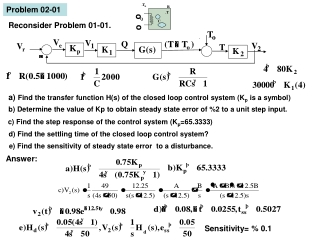DownloadDownload PresentationProblem 02-01

Problem 02-01

Download PresentationProblem 02-01

- - - - - - - - - - - - - - - - - - - - - - - - - - - E N D - - - - - - - - - - - - - - - - - - - - - - - - - - -
Presentation Transcript

1. o o Problem 02-01 Reconsider Problem 01-01. a) Find the transfer function H(s) of the closed loop control system (Kp is a symbol) b) Determine the value of Kp to obtain steady state error of %2 to a unit step input. c) Find the step response of the control system (Kp=65.3333) d) Find the settling time of the closed loop control system? e) Find the sensitivity of steady state error to a disturbance. Answer: Sensitivity= % 0.1

2. Solutions of Week 2: Open-loop system Closed-loop system Problem 02-01: Transfer function between disturbance and output. Transfer function between Reference and Output

3. Solutions of Week 2: v2ss c) Step response of closed loop system v2(t)

4. Solutions of Week 2: The coefficients A and B can be determined by applying Residue Theorem. d) The settling time of the closed loop control system ts The eigenvalue of the closed loop control system is s=-12.5. Then the time constant is calculated as e) Sensitivity of the steady state error to disturbance (The fraction of the steady state error due to disturbance in the form of a unit step function) For unit step disturbance ess=0.001 Sensitivity %0.1 disturbance

5. Problem 02-02 (Kuo, s.195) Reconsider Problem 01-02. a) Find the transfer function of the open loop system [H1(s)]. b) Find the eigenvalues of the open loop system. c) Find the transfer function of the closed loop control system H(s) (Kp is a symbol). d) Find the eigenvalues of the closed loop control system (Kp=9). e) Write the form of the step response of the closed loop control system. f) Determine the steady state error. g) Find the damping ratio, undamped frequency, time increment Δt and settling time. Answer:

6. Solutions of Week 2: Closed system Open system Problem 02-02: a) Transfer function of open loop system b) Eigenvalues of open loop system c) Transfer function of closed loop system c) Eigenvalues of closed loop system for Kp=9. >>roots([10 12 32.59]) s1,2=-0.6±1.7026i

7. Solutions of Week 2: Im ω0 1.7026 α -0.6 Re e) The form of the response of the closed loop system to a unit step input. e) Steady state error : f) Damping ratio, undamped natural frequency, time step (increment) and settling time.

8. Problem 02-03 Reconsider Problem 01-03. a) Find the transfer function of the closed loop control system H(s). (Gc=Kp is a sembol) b) Determine the value of Kp in order to obtain the sensitivity to disturbance as % 4. Answer:

9. Solutions of Week 2: Problem 02-03: K1=0.0034 K2=5.34 Open system Closed System a) Transfer function of closed loop system for Gc(s)=Kp

10. b) Kp value for the sensitivity of 4%.

11. Problem 02-06 Kuo-91 (Sh.389) The step response of a system is given in the figure. Determine a 2nd order tranfer function for this system. Answer: Problem 02-07 Kuo-91 (Sh.389) • Determinethe K andKtvaluesforthesystemwhoseblockdiagram is given in thefigure • Toobtainthemaximumovershoot as 6% andthesteadystateerror as 4.8%. • Findthesteadystateerrorto a unit step input. • WritetheMatlabcode in ordertoplotthe step response of thesystem. Answer:

12. Solutions of Week 2: Problem 02-07: K and Kt values for maximum overshoot 6% and steady state arror 4.8%.

13. Solutions of Week 2: